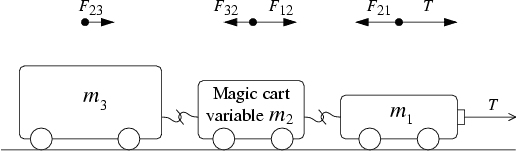# Tension in string in system with multiple objects?## Homework Statement

I am stuck on this problem:
So the question is asking for F21, F12, F32, and F23. (That's the force by 2 on 1).
The mass of m2 is variable.
There are four cases where I need to solve for the forces: when m2 has mass of 3kg, 0.3kg, 0.03kg, and 0kg.
The mass of m1 = 2.5kg, the mass of 3 = 3.5kg.
Also assume that friction is neglected.
I am confused on how to resolved the tension force in a system with numerous objects.
The T is constant, 3N. imagine there's a person pulling on the string with a constant force of 3N.
I know the acceleration of all three carts in each case should be the same.
But how do I solve for the tension force in the strings between the carts?

## Homework Equations

F=ma
Newton's 3rd Law
Pretty sure that's all I really need here.

## The Attempt at a Solution

[/B]Ok, so I drew free-body-diagrams for each case. I know T is always equal to 3N. The weight force and normal force of all three carts aren't taken into account.
Should the tension force in the strings be constant?

Suppose I were to do case 1 with m2 = 3kg:
T=3N
Fnet = 3N
3 = (m1+m2+m3)a
a = 3/(2.5+3+3.5) = 0.33 m/s/s (for the whole system, so each cart has the same acceleration)

Going from left to right, solving for F23, would the force m2 on m3 be equal to (m2+m1)0.33?
Then would F32 be equal in magnitude but in the opposite direction of F23 because of Newton's 3rd Law?

I'm not sure what to include or exclude when I'm calculating a force within a specific part of a system.

Any pointers would be very helpful. Thanks.

Nathanael
Homework Helper
Suppose I were to do case 1 with m2 = 3kg:
T=3N
Fnet = 3N
3 = (m1+m2+m3)a
a = 3/(2.5+3+3.5) = 0.33 m/s/s (for the whole system, so each cart has the same acceleration)
...
Any pointers would be very helpful. Thanks.
I suggest waiting until the end to plug in the values of m2 :)

Should the tension force in the strings be constant?
Yes, because the acceleration is constant. But don't worry about that; it will come out of the math.

Then would F32 be equal in magnitude but in the opposite direction of F23 because of Newton's 3rd Law?
Yes this is true.

I'm not sure what to include or exclude when I'm calculating a force within a specific part of a system.
Okay let's just consider the cart m3. For right now, m3 is our entire system. What is the net force on the system? And how does it relate to acceleration? (Just write "a" for the acceleration for now)

Then treat m2 as your entire system. Write a net force equation for it. Then do m1

Treating each cart as its own system, I'm getting here: (to the right is positive force, to the left is negative force)

The net force acting on m1 is T-F21. Which is equal to 3-(m2a)?
The net force acting on m2 is F12-F32. Which is equal to (m1a)-(m3a)?
The net force acting on m3 is just F23, which is equal to m2a?

And the acceleration is the same for all carts, so the a value should be F/(m1+m2+m3)? Then is it just 3/(m1+m2+m3)?

haruspex
Homework Helper
Gold Member
The net force acting on m1 is T-F21.
Yes.
Which is equal to 3-(m2a)?
T = 3 (N), but why should F21 = m2a?
The net force acting on m2 is F12-F32.
Right, and net force produces acceleration, so according to ##\Sigma F = ma## that net force is equal to ...?

Yes.

T = 3 (N), but why should F21 = m2a?

Right, and net force produces acceleration, so according to ##\Sigma F = ma## that net force is equal to ...?

Ok, so for m2, the Fnet equation would be F12-F32 = m2a2
Then I could solve for a2 by dividing Fnet by m2.
But how could you calculate the individual forces, F12 and F32?

haruspex
Homework Helper
Gold Member
But how could you calculate the individual forces, F12 and F32?
You have three equations, ##\Sigma F = ma## for each of the three masses.
You have three unknowns: two forces and an acceleration.
Solve.

Ok, so for each case I can solve for the acceleration for the entire system.
Then I break up the system into each mass component and treat them independently.

The Fnet of m1 would be T-F21 = m1a

The Fnet of m2 would be F12-F32 = m2a

The Fnet of m3 would be F23 = m3a

I first solve for F21 by using the first equation, then I solve for F32 using the second equation, then I know the F23.

Solved it. Thanks all.